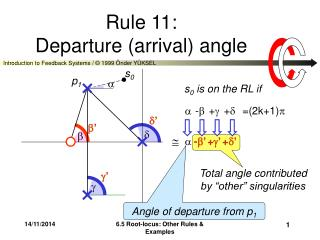DownloadDownload PresentationRule 11: Departure (arrival) angle

# Rule 11: Departure (arrival) angle

Télécharger la présentation## Rule 11: Departure (arrival) angle

- - - - - - - - - - - - - - - - - - - - - - - - - - - E N D - - - - - - - - - - - - - - - - - - - - - - - - - - -
##### Presentation Transcript

1. ’ ’   Total angle contributed by “other” singularities ’  Rule 11:Departure (arrival) angle s0 p1 s0 is on the RL if  - + + =(2k+1)   -’ +’ +’ Angle of departure from p1 6.5 Root-locus: Other Rules & Examples

2. Rule 11 (Cont’d) For the CRL: For the RL: Angle of departure from a pole =(2k+1)π+total angle contributed by “other” singularities Angle of arrival at a zero =(2k+1)π-total angle contributed by “other” singularities 6.5 Root-locus: Other Rules & Examples

3. Example n=4; m=1 # of branches =max(4,1)=4 Starting at 4 poles 1 end at the zero 3 end at infinity 6.5 Root-locus: Other Rules & Examples

4. Example (cont’d) 3 asymptotes at angles: (2k+1)π/3=180o,60o intersecting at: {-3-2-0-0+(-1)}/(4-1)=-2 Real axis loci: Between -2 & 1 & to the left of -3 6.5 Root-locus: Other Rules & Examples

5. Example (cont’d) jω-axis crossing: R-H test yields Single-pole crossing for K=6 Two-pole crossing for K=0 (start) & K=50 at s=j11= j 3.31 6.5 Root-locus: Other Rules & Examples

6. Departure angle from +j1: (2k+1)180o +135 -90 -tan-1(1/2) -tan-1(1/3) =180o 6.5 Root-locus: Other Rules & Examples

7. yields BA points? 3s4+6s3-6s2-14s-11=0 Roots are: 1.6677 -2.2888 -0.6894j0.6966 None on the RL 6.5 Root-locus: Other Rules & Examples

8. COMBINE! 6.5 Root-locus: Other Rules & Examples

9. Check by MATLAB rlocus([1 -1],[1 5 7 5 6]),grid,figure(1) 6.5 Root-locus: Other Rules & Examples

10. Gain for a root to be at s0 Rule 12:Calibration 6.5 Root-locus: Other Rules & Examples

11. z1 z0 |z0-z1| Note: 6.5 Root-locus: Other Rules & Examples

12. Apply to example by MATLAB rlocfind([1 -1],[1 5 7 5 6]), grid,figure(1) 6.5 Root-locus: Other Rules & Examples

13. Examples 6.5 Root-locus: Other Rules & Examples

14. Introduce a zero in LHP rlocus([1 1],[1 0 0]) 6.5 Root-locus: Other Rules & Examples

15. Introduce a 2nd pole in LHP 6.5 Root-locus: Other Rules & Examples

16. Introduce a zero to 2nd order system 6.5 Root-locus: Other Rules & Examples

17. Introduce a pole 6.5 Root-locus: Other Rules & Examples

18. COMPARE 6.5 Root-locus: Other Rules & Examples

19. End of Chapter Restart section Next chapter Restart chapter i The End General index End show 6.5 Root-locus: Other Rules & Examples